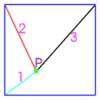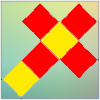# Search by Topic

#### Resources tagged with Pythagoras' theorem similar to Pentabuild:

Filter by: Content type:
Age range:
Challenge level:

### There are 79 results

Broad Topics > Pythagoras and Trigonometry > Pythagoras' theorem### Golden Construction

##### Age 16 to 18 Challenge Level:

Draw a square and an arc of a circle and construct the Golden rectangle. Find the value of the Golden Ratio.### Matter of Scale

##### Age 14 to 16 Challenge Level:

Prove Pythagoras' Theorem using enlargements and scale factors.### Partly Circles

##### Age 14 to 16 Challenge Level:

What is the same and what is different about these circle questions? What connections can you make?### Napkin

##### Age 14 to 16 Challenge Level:

A napkin is folded so that a corner coincides with the midpoint of an opposite edge . Investigate the three triangles formed .### Xtra

##### Age 14 to 18 Challenge Level:

Find the sides of an equilateral triangle ABC where a trapezium BCPQ is drawn with BP=CQ=2 , PQ=1 and AP+AQ=sqrt7 . Note: there are 2 possible interpretations.### Strange Rectangle

##### Age 16 to 18 Challenge Level:

ABCD is a rectangle and P, Q, R and S are moveable points on the edges dividing the edges in certain ratios. Strangely PQRS is always a cyclic quadrilateral and you can find the angles.### Baby Circle

##### Age 16 to 18 Challenge Level:

A small circle fits between two touching circles so that all three circles touch each other and have a common tangent? What is the exact radius of the smallest circle?##### Age 16 to 18

What is the relationship between the arithmetic, geometric and harmonic means of two numbers, the sides of a right angled triangle and the Golden Ratio?### Pythagoras Proofs

##### Age 14 to 16 Challenge Level:

Can you make sense of these three proofs of Pythagoras' Theorem?### Pareq Calc

##### Age 14 to 16 Challenge Level:

Triangle ABC is an equilateral triangle with three parallel lines going through the vertices. Calculate the length of the sides of the triangle if the perpendicular distances between the parallel. . . .### Circle Scaling

##### Age 14 to 16 Challenge Level:

Describe how to construct three circles which have areas in the ratio 1:2:3.### Square Pair Circles

##### Age 16 to 18 Challenge Level:

Investigate the number of points with integer coordinates on circles with centres at the origin for which the square of the radius is a power of 5.### Crescents and Triangles

##### Age 14 to 16 Challenge Level:

Can you find a relationship between the area of the crescents and the area of the triangle?### Nicely Similar

##### Age 14 to 16 Challenge Level:

If the hypotenuse (base) length is 100cm and if an extra line splits the base into 36cm and 64cm parts, what were the side lengths for the original right-angled triangle?### Pythagorean Triples I

##### Age 11 to 16

The first of two articles on Pythagorean Triples which asks how many right angled triangles can you find with the lengths of each side exactly a whole number measurement. Try it!### Pythagorean Triples II

##### Age 11 to 16

This is the second article on right-angled triangles whose edge lengths are whole numbers.### Under the Ribbon

##### Age 14 to 16 Challenge Level:

A ribbon is nailed down with a small amount of slack. What is the largest cube that can pass under the ribbon ?### Picturing Pythagorean Triples

##### Age 14 to 18

This article discusses how every Pythagorean triple (a, b, c) can be illustrated by a square and an L shape within another square. You are invited to find some triples for yourself.### Pythagoras for a Tetrahedron

##### Age 16 to 18 Challenge Level:

In a right-angled tetrahedron prove that the sum of the squares of the areas of the 3 faces in mutually perpendicular planes equals the square of the area of the sloping face. A generalisation. . . .### Little and Large

##### Age 16 to 18 Challenge Level:

A point moves around inside a rectangle. What are the least and the greatest values of the sum of the squares of the distances from the vertices?### Retracircles

##### Age 16 to 18 Challenge Level:

Four circles all touch each other and a circumscribing circle. Find the ratios of the radii and prove that joining 3 centres gives a 3-4-5 triangle.### Two Circles

##### Age 14 to 16 Challenge Level:

Draw two circles, each of radius 1 unit, so that each circle goes through the centre of the other one. What is the area of the overlap?### All Tied Up

##### Age 14 to 16 Challenge Level:

A ribbon runs around a box so that it makes a complete loop with two parallel pieces of ribbon on the top. How long will the ribbon be?### Slippage

##### Age 14 to 16 Challenge Level:

A ladder 3m long rests against a wall with one end a short distance from its base. Between the wall and the base of a ladder is a garden storage box 1m tall and 1m high. What is the maximum distance. . . .### The Fire-fighter's Car Keys

##### Age 14 to 16 Challenge Level:

A fire-fighter needs to fill a bucket of water from the river and take it to a fire. What is the best point on the river bank for the fire-fighter to fill the bucket ?.### Fitting In

##### Age 14 to 16 Challenge Level:

The largest square which fits into a circle is ABCD and EFGH is a square with G and H on the line CD and E and F on the circumference of the circle. Show that AB = 5EF. Similarly the largest. . . .### Some(?) of the Parts

##### Age 14 to 16 Challenge Level:

A circle touches the lines OA, OB and AB where OA and OB are perpendicular. Show that the diameter of the circle is equal to the perimeter of the triangle### Zig Zag

##### Age 14 to 16 Challenge Level:

Four identical right angled triangles are drawn on the sides of a square. Two face out, two face in. Why do the four vertices marked with dots lie on one line?### Rectangular Pyramids

##### Age 14 to 18 Challenge Level:

Is the sum of the squares of two opposite sloping edges of a rectangular based pyramid equal to the sum of the squares of the other two sloping edges?### Chord

##### Age 16 to 18 Challenge Level:

Equal touching circles have centres on a line. From a point of this line on a circle, a tangent is drawn to the farthest circle. Find the lengths of chords where the line cuts the other circles.### The Medieval Octagon

##### Age 14 to 16 Challenge Level:

Medieval stonemasons used a method to construct octagons using ruler and compasses... Is the octagon regular? Proof please.### Medallions

##### Age 14 to 16 Challenge Level:

Three circular medallions fit in a rectangular box. Can you find the radius of the largest one?### Square World

##### Age 16 to 18 Challenge Level:

P is a point inside a square ABCD such that PA= 1, PB = 2 and PC = 3. How big is angle APB ?### 30-60-90 Polypuzzle

##### Age 16 to 18 Challenge Level:

Re-arrange the pieces of the puzzle to form a rectangle and then to form an equilateral triangle. Calculate the angles and lengths.### Kite in a Square

##### Age 14 to 16 Challenge Level:

Can you make sense of the three methods to work out the area of the kite in the square?### Round and Round

##### Age 14 to 16 Challenge Level:

Prove that the shaded area of the semicircle is equal to the area of the inner circle.### Where to Land

##### Age 14 to 16 Challenge Level:

Chris is enjoying a swim but needs to get back for lunch. If she can swim at 3 m/s and run at 7m/sec, how far along the bank should she land in order to get back as quickly as possible?### Holly

##### Age 14 to 16 Challenge Level:

The ten arcs forming the edges of the "holly leaf" are all arcs of circles of radius 1 cm. Find the length of the perimeter of the holly leaf and the area of its surface.### Logosquares

##### Age 16 to 18 Challenge Level:

Ten squares form regular rings either with adjacent or opposite vertices touching. Calculate the inner and outer radii of the rings that surround the squares.### Circle Box

##### Age 14 to 16 Challenge Level:

It is obvious that we can fit four circles of diameter 1 unit in a square of side 2 without overlapping. What is the smallest square into which we can fit 3 circles of diameter 1 unit?### Inscribed in a Circle

##### Age 14 to 16 Challenge Level:

The area of a square inscribed in a circle with a unit radius is, satisfyingly, 2. What is the area of a regular hexagon inscribed in a circle with a unit radius?### The Spider and the Fly

##### Age 14 to 16 Challenge Level:

A spider is sitting in the middle of one of the smallest walls in a room and a fly is resting beside the window. What is the shortest distance the spider would have to crawl to catch the fly?### Pythagoras Mod 5

##### Age 16 to 18 Challenge Level:

Prove that for every right angled triangle which has sides with integer lengths: (1) the area of the triangle is even and (2) the length of one of the sides is divisible by 5.### Three Four Five

##### Age 14 to 16 Challenge Level:

Two semi-circles (each of radius 1/2) touch each other, and a semi-circle of radius 1 touches both of them. Find the radius of the circle which touches all three semi-circles.### Classic Cube

##### Age 16 to 18 Challenge Level:

The net of a cube is to be cut from a sheet of card 100 cm square. What is the maximum volume cube that can be made from a single piece of card?### Incircles Explained

##### Age 16 to 18### Rhombus in Rectangle

##### Age 14 to 16 Challenge Level:

Take any rectangle ABCD such that AB > BC. The point P is on AB and Q is on CD. Show that there is exactly one position of P and Q such that APCQ is a rhombus.### Corridors

##### Age 14 to 16 Challenge Level:

A 10x10x10 cube is made from 27 2x2 cubes with corridors between them. Find the shortest route from one corner to the opposite corner.